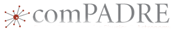# Helping Students Discover Total Internal ReflectionThis material was originally developed through comPADRE
as part of its collaboration with the SERC Pedagogic Service.

## Summary

After students have learned the basic relationship of Snell's Law, they practice applying it to a situation. Then they are given another situation where it "doesn't work." This situation turns out to be one in which total internal reflection occurs. Students are then shown what happens with classroom apparatus.

## Learning Goals

Students will learn
• Snell's Law
• under what circumstances total internal reflection occurs

## Context for Use

Educational level: high school or introductory college
Setting: lecture
Special equipment: Snell's law apparatus

## Description and Teaching Materials

1. Before class, set up the refraction tank. (Several popular choices of refraction tanks are listed in the references and resources section below.)
2. In class, introduce Snell's law in whatever favorite way you have.
3. Give the students a simple problem to solve, such as the one in the box below, involving the materials of your refraction tank.
A beam of light is incident in water and strikes an air-water interface at an incident angle of 15 degrees. What is the angle of refraction?
4. After the students have solved the problem, lead what should hopefully be a brief discussion of the solution. If necessary, have the students direct you while you solve it on the board.
5. Show the class the situation from the problem you just solved with the refraction tank so that they see the answer is indeed correct.
6. Give them another problem to solve where the result is going to be greater than 90 degrees, such as
A beam of light is incident in water and strikes an air-water interface at an incident angle of 50 degrees. What is the angle of refraction?
The students find themselves trying to take the inverse sine of a number greater than 1, which is impossible.
7. Ask the students for some possible explanations for why this might be. Hopefully someone will volunteer the idea that since the index of refraction of the water is greater than that of the air, that the light will make a great angle with the normal in air than in the water. In this case, the angle would be greater than 90 degrees, which seems to indicate that the light will not pass into the air.
8. Show them the situation with the refraction tank and have them observe that the light is totally internally reflected.

## Teaching Notes and Tips

Make sure that you try both situations with the equipment you have in your actual classroom ahead of time, so that you know the results are dramatic enough for the students to see.

## Assessment

Students can be given standard problems involving total internal reflection. For some ideas, you can look at Fundamentals of Physics , 8th edition, Halliday, Resnick, & Walker (Wiley, 2008), chapter 33, problems 57 and 63 (p. 920).

## References and Resources

Refraction Tanks
There are a number of different brands of refraction tanks available. The two links below give you an idea of the basic apparatus. Choose one that will be appropriate for the size of classroom you have.

Science Lab Supplies, item 1084-50, http://www.sciencelabsupplies.com/Complete_Laser_Refraction_Liquid_and_Solid_Apparatus.html

Arbor Scientific, item P2-7090, http://www.arborsci.com/laser-refraction-tank